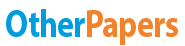Essay by   •  June 15, 2011  •  Essay  •  4,053 Words (17 Pages)  •  1,522 Views

Report this essay
Page 1 of 17

Introduction

In generating probability, it is widely known that the larger the data is, the more reliable the conclusion can be. However, dealing with a large number of pieces of information could lead to misleading conclusion if we are reckless. For instance, there exists invalid intuition among the public that:

If

"females have greater probability of being admitted to the mathematics department of a certain college."

And

"females have greater probability of being admitted to the biology department of a certain college."

Then

"females have greater probability of being admitted to the college assuming that only these two departments recruit new students."

Although such argument seems to be true (in some circumstance it is true), it can be false in other circumstance. Simpson's paradox is just such a "weird" phenomenon. It reveals that when dealing with large data, the trend shown in individual subgroups can reverse that shown in the whole data as a single group.

'Simpson's Paradox refers to the reversal of the direction of a comparison or an association when data from several groups are combined to form a single group' by Moore, D. and McCabe, G.

That is to say, when we analysis the correlation between two or more variables, the results which come from individual partitions of the data may seem to be "conflicted" with result which derived from the data as a whole group. Edward H. Simpson first described this phenomenon in a technical paper in 1951 , but the statisticians Karl Pearson, et al., in 1899, and Udny Yule, in 1903, had mentioned similar effects earlier. The name Simpson's paradox was introduced by Colin R. Blyth in 1972. Other names of Simpson's paradox are Yule-Simpson effect, reversal paradox and amalgamation paradox.

Here is an interesting Ashes Series example presenting Simpson's paradox:

The two Waugh brothers, Steve and Mark, decided to have a little wager on who would have the better overall batting average over the two upcoming Ashes Test series, the first in England and the next here in Australia.

After the first Ashes series finished Steve said to Mark, 'You've got your work cut out for you, mate. I have scored 500 runs for 10 outs, for an average of 50. You have 270 runs for 6 outs, for an average of 45.'

After the second Ashes series, Steve said, 'Ok, mate, pay up. In this series I scored 320 runs for 4 outs, an average of 80, while you had 700 runs for 10 outs, which is only an average of 70. I topped you in each of the Series.'

'Hold on', Mark said, 'The wager was for the better batting average overall, not series by series. As I reckon it, you have scored 820 runs for 14 outs, and I have scored 970 runs for 16 outs. My trusty calculator tells me your average is 58.6, while my average is 60.6. '

In this case, the first and second Ashes series are the partitions (or subgroups) of the whole data. It is clear that Steve could have a better average in each of the two Tests but a lower average overall.

This result may be surprising at first glance, however it is not without logic when we look deep inside.

In the frame of fraction

As I mentioned before, E. H. Simpson discussed a simple fact about fractions that has a wide variety of surprising applications in a seminal paper published in 1951 . The applications arise from the close connections between proportions, percentages, probabilities, and their representations as fractions.

We may have such inequalities for some whole numbers (a, b, c...):

a / b > c / d,

e / f > g / h,

(a + e) / (b + f) < (c + g) / (d + h)

In the Ashes Series example, we can substitute numbers to get the relations the same as above:

500 / 10 > 270 / 6,

320 / 4 > 700 / 10,

(500 + 320) / (10+4) < (270+700) / (6+10)

This algorithm shows that it is possible to get reversal correlation between variables when we consider the data as a group rather than as subgroups. This is because that the addition of fractions is neither simply adding up all the numerators as the new numerator, nor using the sum of denominators as the new denominator. Conversely, the fraction (a + e) / (b + f) in the above expressions usually does not equal to the sum of a/b and e/f. Even though a/b and e/f (probabilities of part of the data) is greater than c/d and g/h respectively, probability of the whole data can reverse (can change probability into fraction to help understand). This fraction algorithm can roughly explain why Simpson's paradox occurs!

However, in the view of fraction algorithm, we only get a blurry idea about the justification of Simpson's paradox. For more in-depth discussion of this topic, we need to view it in terms of conditional probability.

In the frame of conditional probability

Here follows the classical Berkeley admissions case :

An observational study on sex bias in graduate admissions of 1973 was done at the University of California, Berkeley. In the survey, 8442 men and 4321 women applied for the admission, of whom 44% of men and 35% of women were admitted. According to the information above, some claimed that there were sex discriminations against women. In order to discover which department flavor men over women, each department did its own calculation. Individual figure of each department gives puzzle result. Individual figures show that among 6 departments, 4 of them have higher admission rates in women than in men, while only two of the departments have favor in men. The full admission table of 6 departments is as follow:

Department Men Women

...

...